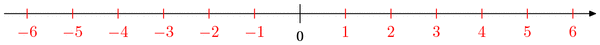Fichier f006.mp (figure 9) — Modifié le 12 Avril 2008 à 10 h 59Source

input newcourbes;
input couleur;
input geometriesyr16;
input TEX;

verbatimtex
%&latex
\documentclass{article}
\usepackage[upright]{fourier}
\usepackage{color}
\def\E{\mathrm{e}}
\let\ve\vec
\def\DR{\mathcal{D}}
\def\CR{\mathcal{C}}
\def\HR{\mathcal{H}}
\newcommand{\Mathbold}{\mbox{\boldmath$#1$\unboldmath}}
\begin{document}
etex
%%%%%%%%%%%%%%%%%%%%%%%%%%%%%%%%%%%%%%%%%%%%%%%%%%%%%%%%%%%%%%
%% aXE

beginfig(9)

repere(0,0,-5,7,-1,1,1cm,1cm);

%r_axes;

drawarrow r_p(-6.5,0)..r_p(6.5,0) ;
end